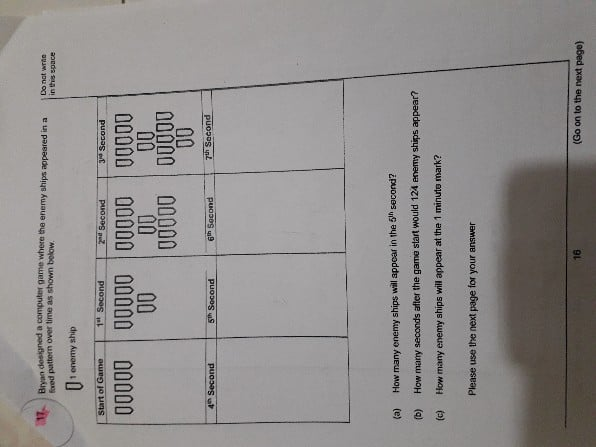QuestionHelp me to solve this question.

Thank u

(a) 3 groups of 7 = 21

(b) At the odd number seconds –> multiples of 7

Number of groups of 7 –> the number of the next second divided by 2

Examples: 3rd second à 4 ÷ 2 = 2, 2 groups of 7

5th second à 6 ÷ 2 = 3, 3 groups of 7

At the even number seconds –> the number of ships for the previous second + 5

Examples: 4th second –> 2 groups of 7 + 5 = 19

6th second –> 3 groups of 7 + 5 = 26

124 is not a multiple of 7, therefore the number belongs to an even number second.

(124 – 5) ÷ 7 = 17

17 x 2 = 34